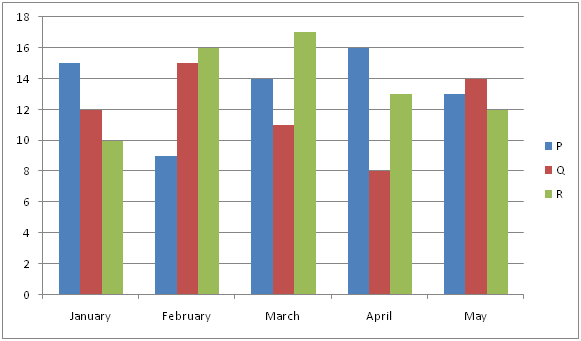# IBPS RRB Clerk/EPFO Assistant/LIC ADO Prelims Quantitative Aptitude Questions 2019 (Day-05)

Dear Aspirants, Our IBPS Guide team is providing new series of Quantitative Aptitude Questions for IBPS RRB Clerk/EPFO Assistant/LIC ADO Prelims 2019 so the aspirants can practice it on a daily basis. These questions are framed by our skilled experts after understanding your needs thoroughly. Aspirants can practice these new series questions daily to familiarize with the exact exam pattern and make your preparation effective.

##### LIC ADO Prelims Free Mock Test
[WpProQuiz 6622]

Directions (Q. 1 – 5): What value should come in place of (?) in the following questions?

1) (3750 ÷ 15) % of 250 = ? + 375

a) 250

b) 325

c) 400

d) 475

e) None of these

2) 24 % of 750 = 28 × 500 ÷ 4 + ? – 4200

a) 720

b) 960

c) 1040

d) 880

e) None of these

3) (3/7) of 315 × 75 ÷ 15 + ? = 729

a) 72

b) 54

c) 66

d) 80

e) None of these

4) 3220 ÷ 14 + 15 × 17 = ? + (13)2

a) 434

b) 482

c) 316

d) 378

e) None of these

5) (29/11) of [6985 – 2365] + 580 = ? + 850

a) 11910

b) 13726

c) 15642

d) 8854

e) None of these

Directions (Q. 6 – 10): Study the following information carefully and answer the given questions?

The following bar graph shows the total savings (In thousands) of 3 different persons in 5 different months.6) Total savings of person P in the month of January and May together is approximately what percentage of total savings of person Q in the month of February and April together?

a) 122 %

b) 135 %

c) 104 %

d) 156 %

e) 88 %

7) Find the difference between the total savings of all the given persons in the month of February to that of April?

a) Rs. 7000

b) Rs. 4000

c) Rs. 3000

d) Rs. 5000

e) None of these

8) Find the ratio between the total savings of person P in the month of February and March together to that of total savings of person R in the month of April and May together?

a) 19 : 24

b) 23 : 25

c) 11 : 17

d) 35 : 46

e) None of these

9) Total savings of person Q and R together in the month of January is approximately what percentage more/less than the total savings of same persons in the month of March?

a) 21 % more

b) 35 % more

c) 35 % less

d) 8 % more

e) 21 % less

10) Find the average savings of person Q in all the given months together?

a) Rs. 14000

b) Rs. 15000

c) Rs. 13000

d) Rs. 12000

e) None of these

Direction (1-5) :

(3750 ÷ 15) % of 250 = x + 375

(3750/15)*(250/100) = x + 375

625 – 375 = x

x = 250

24 % of 750 = 28 × 500 ÷ 4 + x – 4200

(24/100)*750 = (28*500)/4 + x – 4200

180 – 3500 + 4200 = x

x = 880

(3/7) of 315 × 75 ÷ 15 + x = 729

x = 729 – (3/7)*315*(75/15)

x = 729 – 675 = 54

3220 ÷ 14 + 15 × 17 = x + (13)2

(3220/14) + (15*17) – 169 = x

x = 230 + 255 – 169 = 316

(29/11) of [6985 – 2365] + 580 = x + 850

(29/11)*4620 + 580 – 850 = x

x = 12180 + 580 – 850

x = 11910

Direction (6-10) :

Total savings of person P in the month of January and May together

= >15000 + 13000 = Rs. 28000

Total savings of person Q in the month of February and April together

= >15000 + 8000 = Rs. 23000

Required % = (28000 / 23000) * 100 = 122 %

The total savings of all the given persons in the month of February

= >9000 + 15000 + 16000 = Rs. 40000

The total savings of all the given persons in the month of April

= >16000 + 8000 + 13000 = Rs. 37000

Required difference = 40000 – 37000 = Rs. 3000

The total savings of person P in the month of February and March together

= >9000 + 14000 = Rs. 23000

The total savings of person R in the month of April and May together

= >13000 + 12000 = Rs. 25000

Required ratio = 23000 : 25000 = 23 : 25

Total savings of person Q and R together in the month of January

= >12000 + 10000 = Rs. 22000

Total savings of person Q and R together in the month of March

= >11000 + 17000 = Rs. 28000

Required % = [(28000 – 22000) / 28000] * 100 = 21 % less